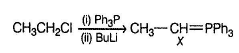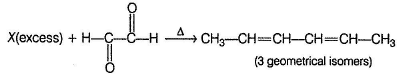Courses

# Test: Reformatsky, Witting Benzil-Benzilic Acid Rearrangements

## 20 Questions MCQ Test Chemistry Class 12 | Test: Reformatsky, Witting Benzil-Benzilic Acid Rearrangements

Description
This mock test of Test: Reformatsky, Witting Benzil-Benzilic Acid Rearrangements for JEE helps you for every JEE entrance exam. This contains 20 Multiple Choice Questions for JEE Test: Reformatsky, Witting Benzil-Benzilic Acid Rearrangements (mcq) to study with solutions a complete question bank. The solved questions answers in this Test: Reformatsky, Witting Benzil-Benzilic Acid Rearrangements quiz give you a good mix of easy questions and tough questions. JEE students definitely take this Test: Reformatsky, Witting Benzil-Benzilic Acid Rearrangements exercise for a better result in the exam. You can find other Test: Reformatsky, Witting Benzil-Benzilic Acid Rearrangements extra questions, long questions & short questions for JEE on EduRev as well by searching above.
QUESTION: 1

### Only One Option Correct Type Direction (Q, Nos. 1-8) This section contains 8 multiple choice questions. Each question has four choices (a), (b), (c) and (d), out of which ONLY ONE is correct. Q.  What is the major product of the following reaction?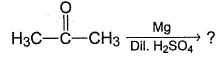Solution:

Free radical coupling reaction of aldehydes and ketones.

QUESTION: 2

### Predict the major product of the following reaction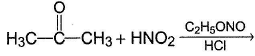Solution:

α-coupling of aldehydes and ketones occur with nitrous acid.

QUESTION: 3

### What is formed as predominant product in the following reaction?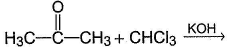Solution: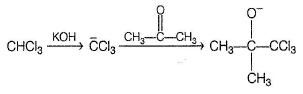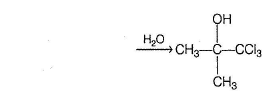QUESTION: 4

Consider the following sequence of reaction,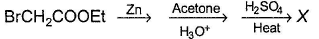Q.

If X is the major organic product, it must be

Solution: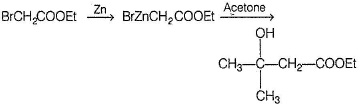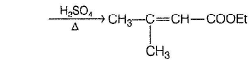QUESTION: 5

Consider the given reaction,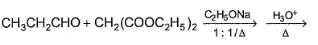Q.

The major organic product is

Solution: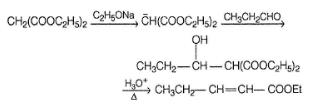QUESTION: 6

In the given reaction,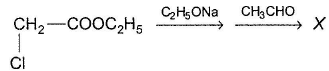Q.

If X is a condensation product then it must be

Solution: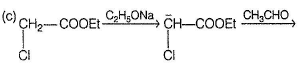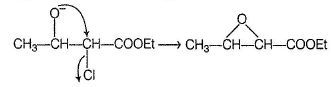QUESTION: 7

Consider the following reaction,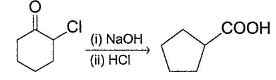Solution: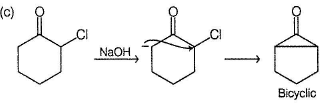QUESTION: 8

In the reaction given below, the final product X is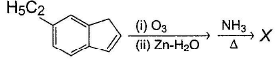Solution: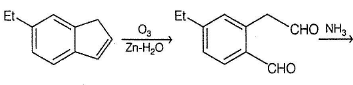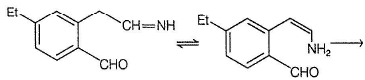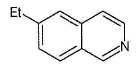*Multiple options can be correct
QUESTION: 9

Direction (Q. Nos. 9-14) This section contains 6 multiple choice questions. Each question has four choices (a), (b), (c) and (d), out of which ONE or MORE THAN ONE am correct.

Consider the following Reformatsky reaction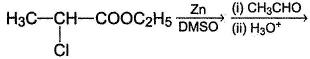Q.

The correct statements concerning the above reaction is/are

Solution: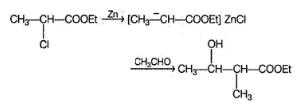*Multiple options can be correct
QUESTION: 10

Consider the following chain reaction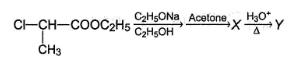Q.

The correct statements concerning the above reaction is/are

Solution: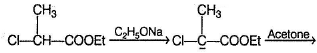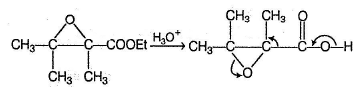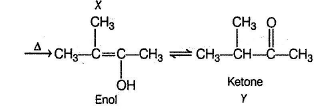*Multiple options can be correct
QUESTION: 11

Consider the following sequence of reaction,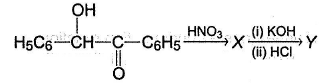Q.

The correct statement(s) regarding the above reaction is/are

Solution:

It is an example of Benzil-benzilic acid rearrangement.

*Multiple options can be correct
QUESTION: 12

Consider the reaction sequence given below,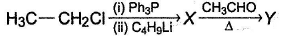Q.

The correct statements regarding the above reaction is/are

Solution:

Wittig reaction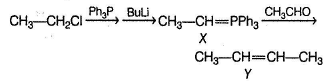Both diastereomers (cis and trans) of Y are formed.

*Multiple options can be correct
QUESTION: 13

Consider the reaction sequence given below,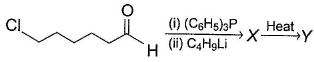Q.

The correct deductions is/are

Solution: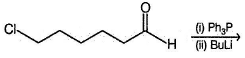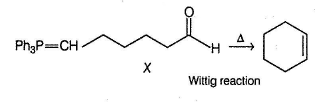*Multiple options can be correct
QUESTION: 14

Consider the following trans formation,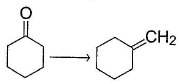Solution: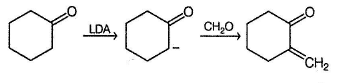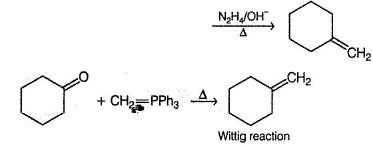QUESTION: 15

Comprehension Type

Direction (Q. Nos. 15-17) This sections contains a paragraph, describing theory, experiments, data, etc.
Three questions related to the paragraph have been given. Each question has only one correct answer among the four given options (a), (b), (c) and (d).

Passage

Benzaldehyde when treated with KCN(aq) undergoes a condensation reaction, known as benzoin condensation forming benzoin A. Benzoin on oxidation with HNO3 gives a diketone known as benzil B. Benzil on heating with ethanolic KOH undergo benzil-benzilic acid rearrangement as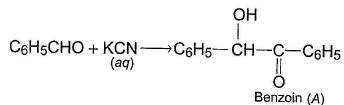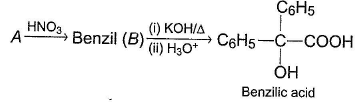The widely accepted mechanism of last step rearrangement is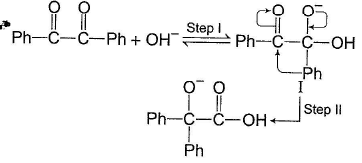Q.

When benzil is treated with dilute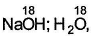both of its oxygens are replaced by O18 isotope. It proves that

Solution: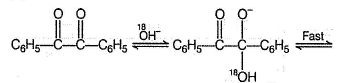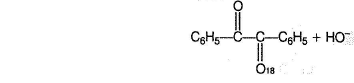QUESTION: 16

Benzaldehyde when treated with KCN(aq) undergoes a condensation reaction, known as benzoin condensation forming benzoin A. Benzoin on oxidation with HNO3 gives a diketone known as benzil B. Benzil on heating with ethanolic KOH undergo benzil-benzilic acid rearrangement asThe widely accepted mechanism of last step rearrangement isQ.

In the following reaction (*C is C14 isotope)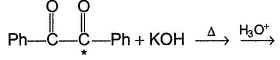The expected organic products is/are

Solution:

Nucleophilic attack at any of the two carbonyl carbons are equally likely.

QUESTION: 17

Benzaldehyde when treated with KCN(aq) undergoes a condensation reaction, known as benzoin condensation forming benzoin A. Benzoin on oxidation with HNO3 gives a diketone known as benzil B. Benzil on heating with ethanolic KOH undergo benzil-benzilic acid rearrangement asThe widely accepted mechanism of last step rearrangement isQ.

In the reaction given below,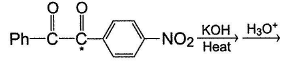The major rearrangement product is

Solution: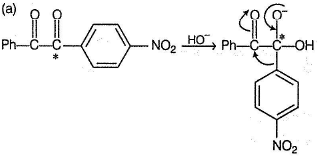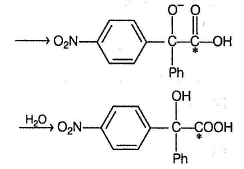*Answer can only contain numeric values
QUESTION: 18

One Integer Value Correct Type

Direction (Q. Nos. 18-20) This section contains 3 questions. When worked out will result in an integer from 0 fo 9 (both inclusive).

Consider the following two step synthesis: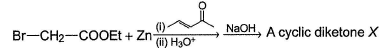Q.

If X is finally treated with excess of NaBH4 followed by acid work-up, how many different isomers of diols would be formed?

Solution: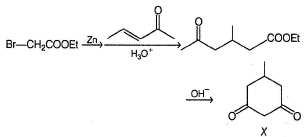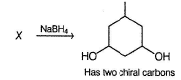*Answer can only contain numeric values
QUESTION: 19

Consider the reaction given below,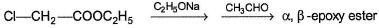Q.

In the above reaction, how many different isomers of esters are formed?

Solution: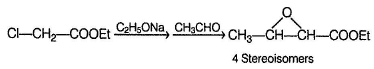*Answer can only contain numeric values
QUESTION: 20

Consider the reaction given below,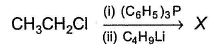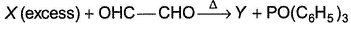Q.

How many different isomeric hydrocarbons of Y are formed?

Solution: